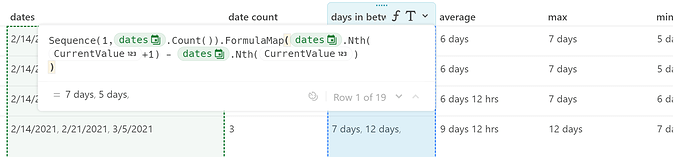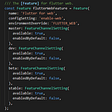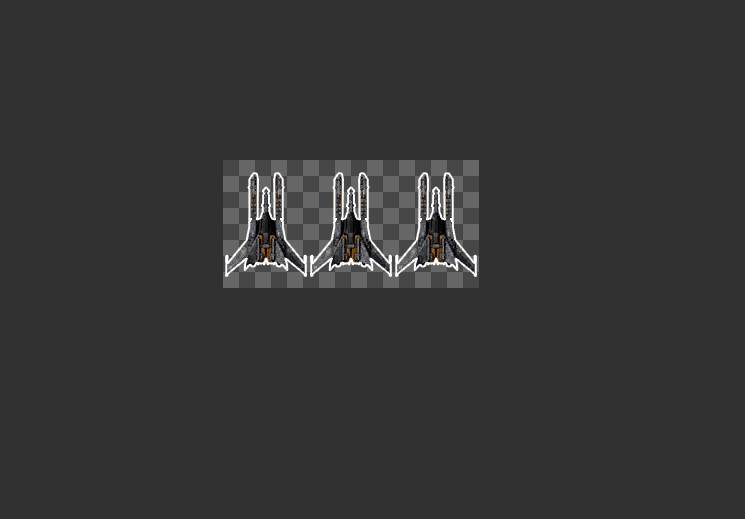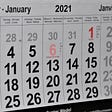# In Coda everything is a list

This blog is about some essentials in Coda I already used in various posts. However if you are new to Coda and you want to speed up your understanding, this might be of help.

• All information in a column can be seen as a list
• In case a table has 10 rows, than any column has 10 items in a list
• If one row is empty, we note `IsBlank( )` it is still an item in this list
• Rows can contain one item (like the date of my birthday) or multiple items (like all the RDV dates for a manager as the outcome of a formula). In any spreadsheet, all cells can only contain one item, not so in Coda, you can have one item or multiples. One or Multiple, we call it an item. This might be confusing if you start using coda. An item in a list can thus contain one or more values.
• Every row represents an item and has a position in the list. We use `Nth()` to get the position in a list of an item. For example on row 3 we find item number 3 (whatever the real content of the item may be) and we note this as `Nth(3)`.
• Since everything in a column is a list and we understand that every item in this list has a position, we can calculate using positions. We can for example look for every third position in a list, thus 1, 3, 6, 9 and so on.
• `CurrentValue` is an item in a list on a certain position. Which position is not the right question because it can be any position. We use an example below to show how it works.
• If we want to have have the value of row 9 in a column about birthdays, we can have something as `BD.Nth(9)`
• The moment you use `Last()`, you have the last position in a list, thus of all the items in a column, `First()` is the first position. `Min()` and `Max()` are not about positions, they are about values like the age of somebody, or weight, or people in a team.

## CurrentValue in action

The formula below I used in this post shows the above logic. The screenshots below show how we put all meeting dates of a person in one cell. We have thus multiple values in one cell (which is not possible in any spreadsheet). Since the intention is to understand if people come back for new meetings we check for days between the meetings. If there are no days, somebody did not come back. The higher the count, the more meetings. First we have to have the days between each date.A table with meetings set and the formula to calcuatle days between dates

This is the formula we applied and it has 4 components

`Sequence(1,thisRow.dates.Count()).FormulaMap( thisRow.dates.Nth(CurrentValue+1) — thisRow.dates.Nth(CurrentValue) )`
• Sequence()
• FormulaMap()
• Nth()
• CurrentValue

Since we need the days between the dates, it follows that we have to execute this calculation as many times as we have dates. We need two tools to make this work. First we have to tell Coda how many times a calculation has to run and second we need a tool that after a first run, starts again to execute a second, a third and so on. To tell Coda how many times we need to run a calculation, we use `Sequence()` and the formula that starts again and again is a `FormulaMap()`

Sequence () gives you a list of numbers between the first number and the last number 1,3 -> 1,2,3 while 8,12 results in 8,9,10,11,12. Both values (start & end) can be hard coded or be the results of an other formula. in our case we start with 1 and we take as second number the amount of dates in the cell ‘dates’. We get this number by counting the dates using : `thisRow.dates.Count()` The outcome is a number like 1, or 2 or 3 and so on and this results in 1 or 2 or 3 calculations. Important to notice is that we assume that the interval is 1 between each number (1,2,3,4,5 etc). However this formula also permits for other intervals like 2 or 3. This interval is standardly one and if we want to have an other value, we put it in the formula as a number or as an outcome of a formula. This is for example the case when you have a team of 5 people and you want to distribute tasks over these people and person 1 always has the first task, team member 2 the second and so on. This is a bit out of scope here. We focus on the function `Sequence()` as a tool to execute multiple calculations.

`Sequence(1,thisRow.dates.Count())`

FormulaMap takes a list as input and runs every item through an expression. Inside the expression, you use CurrentValue to reference the current item being evaluated( Source). What does this somewhat cryptic text mean?

Above we observed that we need to calculate days between dates as many times as we have dates. Our lists contains the numbers we get via `Sequence()` . The expression is the formula we created.

`FormulaMap( thisRow.dates.Nth(CurrentValue+1) — thisRow.dates.Nth(CurrentValue) )`

Before we dive into this, we explain Nth() This is the position in a list. The first date is `Dates.Nth(1) `and the second date is `Dates.Nth(2)` When we want to have the second date minus the first date to find the days between them, we can write `Dates.Nth(2) -` `Dates.Nth(1)` The same for the days between date 3 and date 2: `Dates.Nth(3) -` `Dates.Nth(2)`and so on. It is not so handy to write all these manual functions, when you have 4 dates max, you can do something like this, but if you have many more, it becomes messy and errors slip in easily. Happily the Coda logic supports a smarter way of working.

Instead of typing for every date a number, we make use of `Sequence()` to generate a list of numbers (items) Since each item has a position in the list we combine the real list of dates with the virtual list we created with `Sequence()`. Date 1 is number 1 in the virtual list, Date 2 is number 2 in the virtual list and so forth.

Here we introduce CurrentValue. This is the current item in the list we work with, the item we evaluate. Below you see we inject the virtual number we got via `Sequence()` in the `Nth()` function. Since we do 2 minus 1 and 3 minus 2 and 4 minus 3 we first add 1 with + 1

`thisRow.dates.Nth(CurrentValue+1)`

Now we can put the puzzle together and ask for position (N+ 1) minus N

`FormulaMap( thisRow.dates.Nth(CurrentValue+1) — thisRow.dates.Nth(CurrentValue) )`

The `FormulaMap()` only makes sure we execute the calculation as many times as it finds items in the list we got via `Sequence()` and the CurrentValue is in our case the number in this list. If the list has 3 items, we have three times a CurrentValue, has the list 100 items, we have 100 times a CurrentValue

This brings us back to the main formula which avoids we have to create a manual operation for all dates we got.

`Sequence(1,thisRow.dates.Count()).FormulaMap( thisRow.dates.Nth(CurrentValue+1) — thisRow.dates.Nth(CurrentValue) )`

If you are like me and you do not have any background in coding, be reassured, this is something you can and will learn over time in Coda. Yes it takes a bit of time and you have to get used to a certain logic and order, but once you get the feeling you can create almost any function. I am often surprised how wise my fingers became when I see something happening on the screen not yet fully understanding it, but working!

My name is Christiaan Huizer and I am the owner of Huizer Automation. A company specialised in serving SME in harvesting data and keeping it aligned. I mainly rely on AirTable, Coda, Mailjet, Zapier & Paperform to get the job done. I live in Ghent and serve the BeNelux market.Huizer Automation on how to see everything as a list in Coda.io

--

--

--

## More from Christiaan Huizer

Christiaan uses Coda mainly for planning & calculations of all sorts. Follow me to learn how to Coda with numbers. I blog at least once per week.

Love podcasts or audiobooks? Learn on the go with our new app.

## Meet our supporter: Solana Foundation## How to add a Mac OS User to sudoers## Is Agile Still Relevant?## How Do Laravel Development Services Give a Boost to Your Business?## How to enable Flutter web on the stable channel## Adding to our UI: creating a lives display## 5 Steps To Gaining Control Of Your Cloud Infrastructure Costs## Christiaan Huizer

Christiaan uses Coda mainly for planning & calculations of all sorts. Follow me to learn how to Coda with numbers. I blog at least once per week.

## How to skip over blanks in Coda?## Old doc, new year: Part III## How to Coda with Exchange Rates?## How to Coda contract management?